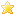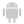Home |Sign in | EnglishHelpWLanguageWLanguage functionsControls, pages and windowsDrawing functionsPrefixed syntaxDrawing functions on WDPic variablesRotationPresentationExampleSee alsoOpenSaveAlphaBlendBackgroundChangeModeDrawArcDrawChordDrawCircleDrawLineDrawPointDrawPolygonDrawPolylineDrawRectangleDrawRectangleGradientDrawRoundedRectangleDrawSliceDrawTextDrawTextRTFEndDrawingFillFontHorizontalSymmetryInvertColorMergeLayerPixelColorPixelOpacityResizeRotationSaveStartDrawingVerticalSymmetryPicMergeLayerPicOpenPicResizePicRotationPicSaveNew WINDEV, WEBDEV 25 feature!
WINDEVWEBDEVWINDEV MobileOthers<WDPic variable>.Rotation (Function)
In french: <Variable WDPic>.Rotation
Rotates a WDPic image. All image layers rotate.
Remark: To rotate one single layer, use <Image>.Rotation.
Example
// Open a source image
MyWDPicImage is WDPic
MyWDPicImage..FileName = fExeDir() + ["\"] + "Source.png"

// Rotate the image
MyWDPicImage.Rotation(45)

// Save the result
MyWDPicImage.Save(fExeDir() + ["\"] + "Result.png")
Syntax

Performing a simple rotation

<Result> = <WDPic image>.Rotation(<Angle> [, <Options>])
<Result>: Boolean
• True if the rotation was performed,
• False otherwise.
<WDPic image>: WDPic variable
Name of the WDPic variable that contains the image to use.
<Angle>: Real
Rotation angle (in degrees).
• If the angle is positive, the image rotates clockwise.
• If the angle is negative, the image rotates anticlockwise.
<Options>: Integer constant
Rotation options:
 drAdapt The image is flipped and enlarged. Then, its size is reduced to correspond to the initial image size. drDefault(default value) Enlarge the image (if necessary) so that it corresponds to the dimensions of rotated image. drNoEnlarging The image is turned but it is not enlarged: the image may be truncated.

Performing a rotation while modifying the rotation center

<Result> = <WDPic image>.Rotation(<Angle> , <X> , <Y> [, <Options>])
<Result>: Boolean
• True if the rotation was performed,
• False otherwise.
<WDPic image>: WDPic variable
Name of the WDPic variable that contains the image to use.
<Angle>: Real
Rotation angle (in degrees).
• If the angle is positive, the image rotates clockwise.
• If the angle is negative, the image rotates anticlockwise.
<X>: Real
New X coordinate of rotation center.
<Y>: Real
New Y coordinate of rotation center.
<Options>: Optional Integer constant
Rotation options:
 drDefault(Default value) Enlarge the image (if necessary) so that it corresponds to the dimensions of rotated image. drNoEnlarging The image is turned but it is not enlarged.
Component : wd250pic.dll
Minimum version required
• Version 25